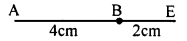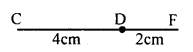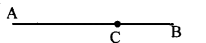# KSEEB Solutions for Class 8 Maths Chapter 3 Axioms, Postulates and Theorems Ex 3.1

Students can Download Maths Chapter 3 Axioms, Postulates and Theorems Ex 3.1 Questions and Answers, Notes Pdf, KSEEB Solutions for Class 8 Maths helps you to revise the complete Karnataka State Board Syllabus and score more marks in your examinations.

## Karnataka Board Class 8 Maths Chapter 3 Axioms, Postulates and Theorems Ex 3.1

Question 1.
What are undefined objects in Euclid’s geometry?
Certain objects in mathematics which cannot be defined using the terms an already know. Lines, points, plane, space are undefined objects in Euclidean geometry.

Question 2.
What is the difference between an axiom and a postulate?
Axiom :

1. Axioms are elementary statements, which are self-evident and which are accepted without questions.
2. Axioms are applicable to all branches of mathematics and science.

Postulate:

1. The statements which are particular to geometry and accepted without question are called geometrical postulates.
2. Postulates are applicable to geometry only.

Question 3.
Give an example of the following axioms from your experience.
a. If equals are added to equals, the wholes are equal.
b. The whole is greater than the part.
a. In the fig. AB = CD = 4cm BE = DF = 2 cmAB + BE = 4 + 2 = 6cm ……….(i)
CD + DF = 4 + 2 = 6cm ……….(ii)From (i) and (ii) AE = CF